## Friday, February 21, 2020

### Photoshop again.

Online seed vendors vary dramatically from the largely respectable, to the folks selling "peppers" like those below that I found on the Amazon or Ebay marketplaces.

To you, the botanically savvy purchaser, these vendors stand out as clearly fraudulent. But not everyone is botanically savvy. People who don't instantly know these wonderful colored peppers are just photoshopped versions of a red pepper photo are such vendors intended "customers".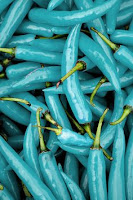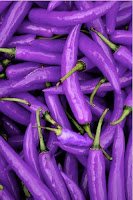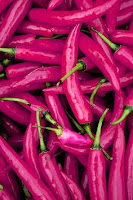Even among the largely respectable vendors, there are a wide range of philosophical or political stances that may impact your decisions of who to buy from. Does the company support white supremacists? Do they sell patented plant varieties? Do they push pseudo-science in their catalogs?

It can take some digging to be certain you agree with the politics behind any given company. It can take significant effort to bring such considerations into your buying decisions, so I understand if you choose not to do so.

But please, don't buy seeds from vendors selling off-hue peppers, blue strawberries, rainbow roses, rainbow onions, or the many other scams that are out there. If something looks too good to be true, at the very least investigate further. These online vendors rely on people clicking "buy" when seeing something interesting. By the time you've grown up the seeds and realize you were scammed, the time to contest the purchase in the marketplaces the vendors work through will have long since expired.

## Friday, February 14, 2020

### Tomatillo Breeding (4/n)

The last couple posts have looked at simulations for selection of a single gene, for recessive or dominant alleles. Increasing the number of genes actively under selection results in it taking longer and longer for the population to converge.
The change in code to simulate multiple genetic loci is really simple if we assume the different alleles we're selecting on are found sufficiently distant from each other on the chromosomes. This is referred to as "un-linked" and means the probability calculations for each are independent of the others.

R Script 5: Multiple recessive traits, large population.
# One recessive trait, infinite population.
#     Stabilize progeny for recessive trait via selection.
#     Save seeds from double-recessive plants each generation.
years <- 10;

# Define F2 population.
P_AA <- vector();
P_Aa <- vector();
P_aa <- vector();
P_AA <- 0.25;
P_Aa <- 0.50;
P_aa <- 0.25;

# Save seeds only from aabb plants, unknown pollen donor. Iterate over years.
for(i in 1:years) {
P_AA <- append(P_AA,   0);
P_Aa <- append(P_Aa,   P_aa[i]*P_AA[i]*1.00 + P_aa[i]*P_Aa[i]*0.50);
P_aa <- append(P_aa,   P_aa[i]*P_aa[i]*1.00 + P_aa[i]*P_Aa[i]*0.50);
P_sum <- P_aa[i+1] + P_Aa[i+1];
P_Aa[i+1] <- P_Aa[i+1]/P_sum;
P_aa[i+1] <- P_aa[i+1]/P_sum;
}

# Make figure.
plot(  0:years, P_aa^1, col="red", main="Multiple recessive traits, large population.", xlab="Years", ylab="%aa pollen donors", xlim=c(0,years), ylim=c(0,1), axes=TRUE, frame.plot=TRUE);
lines(0:years, P_aa^1, col="red");
lines(0:years, 1-P_aa^1, col="blue", lty="dashed");
lines(c(0,years),c(0.95,0.95), col="black", lty="dotted");
lines(c(0,years),c(0.99,0.99), col="black", lty="dashed");

for(i in 2:20) {
lines(0:years, P_aa^i, col="red");
lines(0:years, 1-P_aa^i, col="blue", lty="dashed");
}

R Script 6: Multiple dominant traits, large population.
# One dominant trait, infinite population.
#     Stabilize progeny for dominant trait via selection.
#     Save seeds from dominant plants each generation.
years <- 10;

# Define F2 population.
P_AA <- vector();
P_Aa <- vector();
P_aa <- vector();
P_AA <- 0.25;
P_Aa <- 0.50;
P_aa <- 0.25;

# Save seeds only from (AA and Aa) plants, unknown pollen donor. Iterate over years.
for(i in 1:years) {
P_AA <- append(P_AA,   P_AA[i]*P_AA[i]*1.00 + P_AA[i]*P_Aa[i]*0.50 + P_Aa[i]*P_Aa[i]*0.25);
P_Aa <- append(P_Aa,   P_AA[i]*P_aa[i]*1.00 + P_AA[i]*P_Aa[i]*0.50 + P_Aa[i]*P_aa[i]*0.50 + P_Aa[i]*P_Aa[i]*0.50);
P_aa <- append(P_aa,   0);

P_sum <- P_AA[i+1] + P_Aa[i+1];
P_AA[i+1] <- P_AA[i+1]/P_sum;
P_Aa[i+1] <- P_Aa[i+1]/P_sum;
}

# Make figure.
plot(  0:years, P_AA, col="red", main="Multiple dominant traits, large population.", xlab="Years", ylab="%AA pollen donors", xlim=c(0,years), ylim=c(0,1), axes=TRUE, frame.plot=TRUE);
lines(0:years, P_AA, col="red");
lines(0:years, 1-P_AA, col="blue", lty="dashed");
lines(c(0,years),c(0.95,0.95), col="black", lty="dotted");
lines(c(0,years),c(0.99,0.99), col="black", lty="dashed");

for(i in 2:20) {
lines(0:years, P_AA^i, col="red");
lines(0:years, 1-P_AA^i, col="blue", lty="dashed");
}

The probability of an F2 plant having two copies of recessive alleles for multiple genes drops to minimal very quickly when we increase the number of genes. In a small population this low probability means we might not find an F2 with all the recessive alleles stacked up the way we might want. All is not lost.

With our small F2 population, roughly a quarter would be expected to be in the double-recessive condition for the first gene of interest.

25% AA; 50% Aa; 25% aa

If we were unlucky and couldn't find a single plant that was also double-recessive for the second gene of interest, we can go ahead with plants showing the dominant trait for that second gene. The probability is that two thirds of the plants showing the dominant trait for the second gene will be heterozygous, carrying one copy of the recessive allele.

aaB_ (⅓BB; ⅔Bb)

In the next generation we have pretty good odds of recovering that second recessive trait that we were looking for. This way we can progressively collect multiple recessive traits without finding them in that first F2 generation. With this strategy, we need to keep seeds from prior generations. If we can't recover that next recessive trait in the next year, then we managed to find plants that were not heterozygous for the gene of interest. We need to grow more plants from the previous generation again, to try and find some carrying a copy of the recessive allele.

With plants that typically self-pollinate (like peppers and tomatoes), it can be pretty simple to intentionally remove recessive alleles for genes of interest. If you grow out the seeds produced by a plant and find any double-recessive progeny, you know that plant was heterozygous. If you don't find any double-recessive progeny, if you grow enough seeds, you can be pretty confident of that plant being homozygous for the dominant allele.

With plants that can't self-pollinate (like tomatillos), it can take more work/time. Lets say we have one plant that is showing the dominant trait. If we cross it with a plant showing the recessive trait, the resulting progeny will tell us if that first plant is "AA" or "Aa". If all the progeny show the dominant trait, then the plant we were testing is "AA". If the progeny show a mix of dominant and recessive traits, then the plant we were testing is "Aa" (and can be discarded). This is called a "test-cross" because it is used to test the genetics of a specific individual, even though we have no interest in using the progeny that result for further breeding work.

Since tomatilloes can be kept alive over several years, you can use such test crosses to progressively collect multiple plants with just the dominant alleles for your genes of interest. Once you have a few such plants, you can then allow them to inter-cross and be confident you won't have the recessive allele turning up in the next generations.

## Friday, February 7, 2020

### Tomatillo Breeding (3/n)

I've been doing some math to help me think about breeding strategies with tomatillos. Last week I showed some code for calculating how populations of different sizes converge under selection for a single recessive trait. Here I'll show similar code for a single dominant trait.Solid red curve with circles: %AA pollen donors. Dashed blue curve: %Aa & %aa pollen donors.

Since we can't tell the difference between plants with one or two copies of the dominant trait ("AA" or "Aa"), we can't tell what the genetic status is of any one plant that we save seeds from. Our goal is a population entirely consisting of "AA" plants, so that is what the code will plot.

The zero year is our F2 population. It takes seven years for the "AA" individuals to represent 95% (dotted horizontal line) of the population. Three years later the level crosses above 99% (dashed horizontal line) of the population.

Because this is the infinite population scenario, there will always be a small percentage of the population carrying the recessive allele.

R Script 3: One dominant trait, infinite population.
# One dominant trait, infinite population.
#     Stabilize progeny for dominant trait via selection.
#     Save seeds from dominant plants each generation.
years <- 10;

# Define F2 population.
P_AA <- vector();
P_Aa <- vector();
P_aa <- vector();
P_AA <- 0.25;
P_Aa <- 0.50;
P_aa <- 0.25;

# Save seeds only from (AA and Aa) plants, unknown pollen donor. Iterate over years.
for(i in 1:years) {
P_AA <- append(P_AA,   P_AA[i]*P_AA[i]*1.00 + P_AA[i]*P_Aa[i]*0.50 + P_Aa[i]*P_Aa[i]*0.25);
P_Aa <- append(P_Aa,   P_AA[i]*P_aa[i]*1.00 + P_AA[i]*P_Aa[i]*0.50 + P_Aa[i]*P_aa[i]*0.50 + P_Aa[i]*P_Aa[i]*0.50);
P_aa <- append(P_aa,   0);

P_sum <- P_AA[i+1] + P_Aa[i+1];
P_AA[i+1] <- P_AA[i+1]/P_sum;
P_Aa[i+1] <- P_Aa[i+1]/P_sum;
}

# Make figure.
plot(  0:years, P_AA, col="red", main="One dominant trait, large population.", xlab="Years", ylab="%AA pollen donors", xlim=c(0,years), ylim=c(0,1), axes=TRUE, frame.plot=TRUE);
lines(0:years, P_AA, col="red");
lines(0:years, P_Aa+P_aa, col="blue", lty="dashed");
lines(c(0,years),c(0.95,0.95), col="black", lty="dotted");
lines(c(0,years),c(0.99,0.99), col="black", lty="dashed")Cyan line w/circles: recessive selection. Red line w/circles: dominant selection.
To compare the trajectory for selection on the recessive allele vs on the dominant allele, I overlaid the two curves in an image editor. I inverted the colors for the recessive curves to better distinguish them from the added dominant curves.

Selection on a dominant trait progresses at a slower rate initially than selection on a recessive trait, but by about ten years the two approaches would be expected to reach a similar degree of completeness.

With smaller population sizes, we'd expect the selected allele (dominant or recessive) to reach complete saturation by about that time point.

With recessive traits, I only had to consider "aa" plants as seed producers. With dominant traits, I have to consider "AA" and "Aa" plants. This seems like a small difference, but for simulating small numbers this adds significant complexity.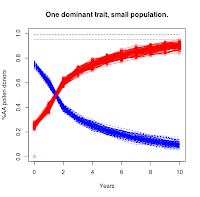Population = 1000Population = 50Population = 10

If you compare these plots to those for the recessive selection scenario (https://the-biologist-is-in.blogspot.com/2020/01/tomatillo-breeding-2n.html), you'll see that this scenario has a much higher level of noise in the trajectories. For the smallest population level, it takes 30 years (not shown in figures) for the majority of the experimental replicates to converge on the targeted "AA" condition.

R Script 4: One dominant trait, small population.
# One dominant trait, small population.
#     Stabilize progeny for dominant trait via selection.
#     Save seeds from dominant plants each generation.
years <- 10;
population <- 1000; # 1000, 50, 10
trials <- 100;

# Intialize figure.
plot( c(0,years),c(0,years), col="red", main="One dominant trait, small population.", xlab="Years", ylab="%AA pollen donors", xlim=c(0,years), ylim=c(0,1), axes=TRUE, frame.plot=TRUE);
lines(c(0,years),c(0.95,0.95), col="black", lty="dotted");
lines(c(0,years),c(0.99,0.99), col="black", lty="dashed");

for (ii in 1:trials) {
# Define F2 population probabilities for selection on AA plants.
P_AA_1 <- vector();
P_Aa_1 <- vector();
P_aa_1 <- vector();
P_AA_1 <- 0.25;
P_Aa_1 <- 0.50;
P_aa_1 <- 0.25;

# Define F2 population probabilities for selection on Aa plants.
P_AA_2 <- vector();
P_Aa_2 <- vector();
P_aa_2 <- vector();
P_AA_2 <- 0.25;
P_Aa_2 <- 0.50;
P_aa_2 <- 0.25;

# Save seeds only from (AA and Aa) plants, which can't self-polinate.
for (i in 1:(years+2)) {
# Generate actual population.
rands <- runif(population, 0, 1);
Genotypes <- vector();
for (j in 1:population) {
if (rands[j] < P_AA_1[i]) {
Genotypes <- append(Genotypes, "AA");
} else if (rands[j] < P_AA_1[i]+P_Aa_1[i]) {
Genotypes <- append(Genotypes, "Aa");
} else {
Genotypes <- append(Genotypes, "aa");
}
}
Genotype_counts <- table(Genotypes);

# Determine actual genotype probabilities for pollen donors. (Assuming "AA" plant in case 1, "Aa" plant in case 2.)
if (is.na(Genotype_counts["AA"])) {
P_AA_1[i] <- 0;
P_AA_2[i] <- 0;
} else {
P_AA_1[i] <- (Genotype_counts["AA"]-1)/(population-1); # The plant we're saving seeds from can't be polinated by itself.
P_AA_2[i] <- Genotype_counts["AA"]/(population-1);
}
if (is.na(Genotype_counts["Aa"])) {
P_Aa_1[i] <- 0;
P_Aa_2[i] <- 0;
} else {
P_Aa_1[i] <- Genotype_counts["Aa"]/(population-1);
P_Aa_2[i] <- (Genotype_counts["AA"]-1)/(population-1); # The plant we're saving seeds from can't be polinated by itself.
}
if (is.na(Genotype_counts["aa"])) {
P_aa_1[i] <- 0;
P_aa_2[i] <- 0;
} else {
P_aa_1[i] <- Genotype_counts["aa"]/(population-1);
P_aa_2[i] <- Genotype_counts["aa"]/(population-1);
}

# Generate new theoretical genotype probabilities.
P_AA_1 <- append(P_AA_1,   P_AA_1[i]*P_AA_1[i]*1.00 + P_AA_1[i]*P_Aa_1[i]*0.50 + P_Aa_1[i]*P_Aa_1[i]*0.25);
P_Aa_1 <- append(P_Aa_1,   P_AA_1[i]*P_aa_1[i]*1.00 + P_AA_1[i]*P_Aa_1[i]*0.50 + P_Aa_1[i]*P_aa_1[i]*0.50 + P_Aa_1[i]*P_Aa_1[i]*0.50);
P_aa_1 <- append(P_aa_1,   0);

P_AA_2 <- append(P_AA_2,   P_AA_2[i]*P_AA_2[i]*1.00 + P_AA_2[i]*P_Aa_2[i]*0.50 + P_Aa_2[i]*P_Aa_2[i]*0.25);
P_Aa_2 <- append(P_Aa_2,   P_AA_2[i]*P_aa_2[i]*1.00 + P_AA_2[i]*P_Aa_2[i]*0.50 + P_Aa_2[i]*P_aa_2[i]*0.50 + P_Aa_2[i]*P_Aa_2[i]*0.50);
P_aa_2 <- append(P_aa_2,   0);

P_sum_1 <- P_AA_1[i+1] + P_Aa_1[i+1];
P_AA_1[i+1] <- P_AA_1[i+1]/P_sum_1;
P_Aa_1[i+1] <- P_Aa_1[i+1]/P_sum_1;

P_sum_2 <- P_AA_2[i+1] + P_Aa_2[i+1];
P_AA_2[i+1] <- P_AA_2[i+1]/P_sum_2;
P_Aa_2[i+1] <- P_Aa_2[i+1]/P_sum_2;

# Weighted average of the two probability sets by proportion of "AA" vs "Aa" plants.
#  Only _1 values carry over to next iteration.
if (is.na(Genotype_counts["AA"])) {
count_AA <- 0; } else {
count_AA <- Genotype_counts["AA"];
}
if (is.na(Genotype_counts["Aa"])) {
count_Aa <- 0; } else {
count_Aa <- Genotype_counts["Aa"];
}
weight1 <- count_AA/(count_AA+count_Aa);
weight2 <- 1-weight1;
val_AA_1 <- P_AA_1[i+1];
val_AA_2 <- P_AA_2[i+1];
val_Aa_1 <- P_Aa_1[i+1];
val_Aa_2 <- P_Aa_2[i+1];
P_AA_1[i+1] <- val_AA_1*weight1 + val_AA_2*weight2;
P_Aa_1[i+1] <- val_Aa_1*weight1 + val_Aa_2*weight2;

if (is.na(P_AA_1[i+1]) == TRUE) {  P_AA_1[i+1] <- 0;  }
if (is.na(P_Aa_1[i+1]) == TRUE) {  P_Aa_1[i+1] <- 0;  }

if ((P_AA_1[i+1]+P_Aa_1[i+1]) == 0) {
# End simulation cycle if no "AA" or "Aa" plants.
for (j in (length(P_aa_1)):years) {
P_AA_1 <- append(P_AA_1,   0);
P_Aa_1 <- append(P_Aa_1,   0);
P_aa_1 <- append(P_aa_1,   0);
}
break;
}

## Debugging output.
#message("Iteration ", i);
#print(Genotypes);
#message("  ");
}

# Add current simulation cycle to figure.
points(0:years, P_AA_1[1:(years+1)], col="red");
lines( 0:years, P_AA_1[1:(years+1)], col="red");
lines( 0:years, 1-P_AA_1[1:(years+1)], col="blue", lty="dashed");
}

This essentially means it isn't possible to selectively breed a dominant trait to complete saturation in a small population just using simple selection.

Unlike in the recessive case, we can't just save a few plants over winter to reset the population with only the exact genetics we want. A similar strategy should allow for more rapid progress towards the goal, however.

I'll explore this topic further next time.

## Friday, January 31, 2020

### Tomatillo Breeding (2/n)

I thought it would take me a week to get back to this, but that didn't happen. Oops. Sorry.

The big difficulty with tomatillo breeding is that they're very strong out-crossers. Unlike tomatoes, peppers, eggplant, beans, etc., you can't just grow one plant from each generation to help reduce control the genetics during the process of making a new variety. If you grow a dozen tomatillo plants and don't like how half of them grew, you can be sure that seeds saved from the plants you liked will have genetics from the ones you didn't.

I worked out some of the math long-hand, showing how this difficulty plays out over several generations. I faltered when it came to the task of outlining all those calculations via text. It is easy enough to throw a few equations into text, but I didn't want to post pages of derivations for you to read through. (And I'd have most assuredly made silly typos along the way.)

Instead, I wrote up some simulations in R. These can be run using RStudio if you want to play around with them, or you can just look at my summary figures here.Solid red curve with circles: %aa pollen donors. Dashed blue curve: %Aa & %AA pollen donors.
We'll start with the simple case of a single recessive trait in an infinite population. (Sometimes infinity makes the math hard to do, other times it makes it very easy.)

If we seeds only from plants showing the recessive trait, we can rapidly select away the dominant allele. The zero year of this plot is the F2 generation, where traits first start assorting. It takes five years for the recessive trait to be at 95% (dotted horizontal line) of the population and another three for it to be at 99% (dashed horizontal line) of the population.

Because the population is infinite, we can never quite reach 100%. There will always be a small amount of the dominant allele hanging around.

R Script 1: One recessive trait, infinite population.
# One recessive trait, infinite population.
#     Stabilize progeny for recessive trait via selection.
#     Save seeds from double-recessive plants each generation.
years <- 10;

# Define F2 population.
P_AA <- vector();
P_Aa <- vector();
P_aa <- vector();
P_AA <- 0.25;
P_Aa <- 0.50;
P_aa <- 0.25;

# Save seeds only from aa plants, unknown pollen donor. Iterate over years.
for(i in 1:years) {
P_AA <- append(P_AA,   0);
P_Aa <- append(P_Aa,   P_aa[i]*P_AA[i]*1.00 + P_aa[i]*P_Aa[i]*0.50);
P_aa <- append(P_aa,   P_aa[i]*P_aa[i]*1.00 + P_aa[i]*P_Aa[i]*0.50);
P_sum <- P_aa[i+1] + P_Aa[i+1];
P_Aa[i+1] <- P_Aa[i+1]/P_sum;
P_aa[i+1] <- P_aa[i+1]/P_sum;
}

# Make figure.
plot(  0:years, P_aa, col="red", main="One recessive trait, large population.", xlab="Years", ylab="%aa pollen donors", xlim=c(0,years), ylim=c(0,1), axes=TRUE, frame.plot=TRUE);
lines(0:years, P_aa, col="red");
lines(0:years, P_Aa+P_AA, col="red", lty="dashed");
lines(c(0,years),c(0.95,0.95), col="black", lty="dotted");
lines(c(0,years),c(0.99,0.99), col="black", lty="dashed")


We can extend this simulation to better model a realistic situation where you can only grow a limited number of plants. Coding this is much more complicated. If we run it with a large population, we see a pattern much like the infinite one above. If we run it with a small population, we get very noisy trajectories that vary a lot from run to run. At small population numbers, it is fairly easy to accidentally end the experiment with no "aa" plants to save seeds from. (In real life, we'd just go back to seeds from the previous generation.)Population = 1000Population =50Population =10

The upshot of the simulations is that if we grow small numbers of plants each generation, we can eventually eliminate the pesky dominant alleles for the trait of interest. It will take a while, but it is doable if you're willing to wait several years to a decade.

R Script 2: One recessive trait, small population.
# One recessive trait, small population.
#     Stabilize progeny for recessive trait via selection.
#     Save seeds from double-recessive plants each generation.
years <- 10;
population <- 50; # 1000, 50, 10
trials <- 100;

# Intialize figure.
plot(  c(0,years),c(0,years), col="red", main="One recessive trait, small population.", xlab="Years", ylab="%aa pollen donors", xlim=c(0,years), ylim=c(0,1), axes=TRUE, frame.plot=TRUE);
lines(c(0,years),c(0.95,0.95), col="black", lty="dotted");
lines(c(0,years),c(0.99,0.99), col="black", lty="dashed");

for (ii in 1:trials) {
# Define F2 population probabilities
P_AA <- vector();
P_Aa <- vector();
P_aa <- vector();
P_AA <- 0.25;
P_Aa <- 0.50;
P_aa <- 0.25;

# Save seeds only from "aa" plants, which can't self-polinate.
for (i in 1:(years+2)) {
# Generate actual population.
rands <- runif(population, 0, 1);
Genotypes <- vector();
for (j in 1:population) {
if (rands[j] < P_AA[i]) {
Genotypes <- append(Genotypes, "AA");
} else if (rands[j] < P_AA[i]+P_Aa[i]) {
Genotypes <- append(Genotypes, "Aa");
} else {
Genotypes <- append(Genotypes, "aa");
}
}
Genotype_counts <- table(Genotypes);

# Determine actual genotype probabilities for pollen donors.
if (is.na(Genotype_counts["AA"])) {
P_AA[i] <- 0; } else {
P_AA[i] <- Genotype_counts["AA"]/(population-1);
}
if (is.na(Genotype_counts["Aa"])) {
P_Aa[i] <- 0; } else {
P_Aa[i] <- Genotype_counts["Aa"]/(population-1);
}
if (is.na(Genotype_counts["aa"])) {
P_aa[i] <- 0; } else {
P_aa[i] <- (Genotype_counts["aa"]-1)/(population-1); # The plant we're saving seeds from can't be polinated by itself.
}

# Generate new theoretical genotype probabilities.
P_AA <- append(P_AA,   0);
P_Aa <- append(P_Aa,   P_aa[i]*P_AA[i]*1.00 + P_aa[i]*P_Aa[i]*0.50);
P_aa <- append(P_aa,   P_aa[i]*P_aa[i]*1.00 + P_aa[i]*P_Aa[i]*0.50);

P_sum <- P_aa[i+1] + P_Aa[i+1];
P_Aa[i+1] <- P_Aa[i+1]/P_sum;
P_aa[i+1] <- P_aa[i+1]/P_sum;

if (is.na(P_Aa[i+1]) == TRUE) {  P_Aa[i+1] <- 0;  }
if (is.na(P_aa[i+1]) == TRUE) {  P_aa[i+1] <- 0;  }

if (P_aa[i+1] == 0) {
# End simulation cycle if no "aa" plants.
for (j in (length(P_aa)):years) {
P_AA <- append(P_AA,   0);
P_Aa <- append(P_Aa,   0);
P_aa <- append(P_aa,   0);
}
break;
}
}

# Add current simulation cycle to figure.
points(0:years, P_aa[1:(years+1)], col="red");
lines( 0:years, P_aa[1:(years+1)], col="red");
lines( 0:years, 1-P_aa[1:(years+1)], col="blue", lty="dashed");
}

However, there's a much faster way to complete the process. It might even only take a couple years.

Tomatilloes are perennial where it is warm enough for them to survive through winter. They also easily root from cuttings. These traits combined mean we can pot up rooted cuttings from each plant at the end of one year and continue growing selected plants the next year after we've had a chance to evaluate their fruit characteristics.

You'd have to grow enough plants the first year to be able to find multiple individuals with the recessive traits you're interested in. The next year, you can continue growing only those few plants and allow them to cross-pollinate. Every seed they produce in their second year will contain those recessive traits you selected the parents for. The dominant alleles will be gone from your population.

You're done in one year of selection and another for seed production. No waiting around for a decade or more, gambling with the whims of chance. You may have your new tomatillo variety complete and ready to go.

I didn't talk about dominant traits here. They're a bit more involved and I'll have to do another post about that case. I'll also have to do another post talking about the case where you're looking for multiple specific genes (recessive or dominant) at once.

It looks like my planned two part series is going to be a bit longer in the end.

## Thursday, December 5, 2019

### Tomatillo Breeding (1/n)

Tomatillos are a wonderful vegetable plant to grow. There are several distinct varieties available, but nowhere near the numbers we see for tomatoes, peppers, or other crops. What's the difference?

Tomatillos are almost exclusively out-breeders. You need two or more plants growing in an area to get good production of fruit. As a result, every plant is a new hybrid and a population will maintain a high degree of genetic diversity. This also makes it difficult for different varieties to be grown in the same area, as they will generally cross and meld into one diverse population.

A few years back I started an experiment with breeding tomatillos. I grew one plant of a variety with small purple fruit next to one plant of a variety with large green fruit. I had saved seeds from a CSA and the local grocer, so I don't have any specific variety names to give you. (If you want to replicate the experiment, the purple variety was similar to: https://www.edenbrothers.com/store/purple-tomatillo-seeds.html; the green to: https://www.edenbrothers.com/store/rio-grande-verde-tomatillo-seeds.html.)#1. Medium purple fruit. #2. Small purple fruit. #3. Large green fruit, with purple dots. #4. Medium purple fruit.
Because the plants are such extreme out-crossers, every seed that year was expected to be a hybrid between the two different varieties. The next year I grew four plants, from seeds I saved from the purple plant. Each plant grew distinct fruit. (1-4, left to right in photo at right.) This diversity tells us that both parental varieties were highly heterogeneous, so the specifics of each hybrid plant depended on exactly which allele they inherited from each parent. As none of my neighbors were growing tomatillos, we can be pretty sure each one was pollinated by the other three.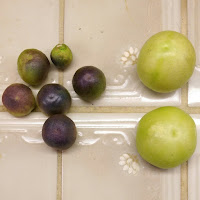F2s from F1#3.

The next year I planted seeds I had saved from plant #3. I grew 11 plants, but only 5 produced any fruit. The plants looked like they'd been exposed to an herbicide from the commercial garden soil I had added to the garden at the start of the season (Herbicide carryover). All the fruit were green, with some later developing some purple pigment as they ripened off the plant.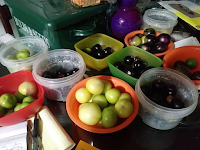F2s from F1#4.
This year I planted seeds I had saved from plant #4. I grew 12 plants and all produced fruit. These showed a much wider range of pigment levels, including a pair of plants with visible purple pigment and large fruit.

One plant had a trait I didn't like at all. The fruit from spoiled very rapidly after picking. (Previous year's fruit stored for months.) That plant was one of two in an isolated garden, so I immediately culled all of the fruit from both plants. I didn't want to risk the genetics associated with spoilage turning up in the garden again next year.

One plant had fruit I really liked. The fruit were large and developed purple pigment, the traits I have been trying to combine in one plant. I wasn't expecting the fruit to develop stripes as they were maturing, however. These fruit are not lasting as long as I'd like, but the other good traits means I'll be saving seeds from them anyhow.A couple other plants produced intensely dark purple fruit, appearing ink-black. This is the color I've been looking for, but the fruit aren't as large as I want. I'll save seeds from these as well.

Because the plants are out-crossers, I know they will have been pollinated by the others in the garden. Even though these two have trait combinations I really like, it will be unlikely to find offspring with the same traits because of all the other traits in the garden.

I've tried to diagram the overall history of the project so far. (I didn't have any photos of the original varieties, so they get cartoon representations.)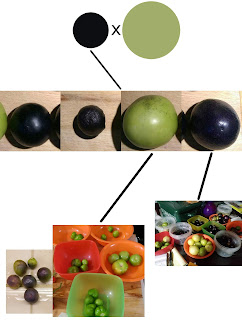Tomatillo project history so far.

About this point I started thinking about how I might get around the issues caused by the potential for genes from every plant in a garden to turn up in the next generation. I don't want to have to cull everything from a garden when something strongly negative turns up in the population. Right now I only have two isolated garden spaces, so that strategy can only go so far.

For my solution, come back in a week for part 2!

References:

## Thursday, November 28, 2019

### Fava Beans[Photo from link.]
I've eaten fava beans (Vicia faba) from time to time, but I've never grown them. I was recently perusing some postings from blogs I occasion and found an interesting post. The post contains a wonderful series of photos of fava bean flowers in the author's garden, ranging in shades of red/pink and brown/black. A forum discussion revealed that these variations were the result of crossing the varieties "Crimson Flowered" and "Red Epicure". After searching around a bit, I found that for the vast majority of fava bean varieties the flowers are only red/pink or brown/black.

The flowers are impressive enough in the garden already. Some improvement in flower size or color range would be awesome. My biology background leads me to think of at least two strategies.
1. Hybridize F. faba with related species with different flower colors.
2. Find rare varieties of F. faba with different colors.
1. Hybridization? I like this strategy generally, but the usefulness of the strategy depends on the plant being worked with. It turns out that there are no known species which can be used to produce hybrid seed with V. faba. There is some research looking into why crosses don't work. F. faba as seed parent crossed with V. galilaea and V. johannis both appear to result in fertilized eggs. F. faba as pollen parent crossed with V. bithynica also appears to result in fertilized eggs. The fertilized eggs don't seem to result in viable seeds, however. There is some later developmental failure which interferes with the cross. It might be possible to use embryo rescue to allow some of those crosses to grow up. This is well outside my skill set for now.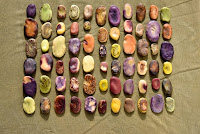[Photo from link.]
2. Finding old/rare varieties relies on such varieties still existing. The internet provides us with an amazing ability to find things, so long as someone, somewhere has put it online in some form. The image at right and others suggest there's a great deal of genetic diversity around, which might include interesting traits impacting flower color. The task of getting seeds to trial may be rather involved, but it is definitely a way forward.

References:

## Thursday, November 21, 2019

### The Color of Onions : The Whims of Genetics

I've previously posted about what might go into changing the color of onions (the-biologist-is-in.blogspot.com/2013/12/the-color-of-onions.html), but now I've gone and done an experiment. It was an accident, really, but many useful experiments start out as accidents.

We planted out a batch of "red" onion seedlings this last spring. We got them in a trade from someone who had started them. We got a pot of onion threads, and they got a couple squash babies in return. I'd never grown onions before, so I just put them (along with several types of decorative onions, hoping the deer would leave them all alone) into a raised bed that I had recently cleared. I watered the babies a few times when I noticed the soil was dry. I never fertilized or amended the soil. I basically ignored them. In retrospect, this is not the way to get those luxuriant onions you see in the store. Somehow, almost all the plants survived and produced bulbs. Inch-long bulbs, that is.

I pulled each onion as its leaves died down. They got cleaned, dried, and then left alone on the kitchen windowsill. After too many had accumulated, I moved them to a spare drying rack left over from an ongoing tomato-jerky experiment with a food dehydrator. A couple days later, I happened to notice that one of the bulbs was a much darker color than all the others. An early thought was that this was the color of some mold infesting the bulb, but on close examination there didn't seem to be anything wrong with it. It definitely was a darker shade.

I started looking at the color of the collected onions. One stood out as being more red than the others... actually red instead of that purplish color that "red" onions typically are. Another was a rich purple color.www.braukaiser.com/wiki/index.php?title=An_Overview_of_pH
Since "red" onions are colored by anthocyanins that change their color depending on pH, we can estimate the pH of the cellular structures where the pigment is found. The red bulb approaches a pH of 2, while the purple bulb approaches a pH of 5. If we could drive the pH further to the right by the same interval, we'd get a pH=8 onion that looked blue.

I was planning to save the color outlier bulbs (red, purple, dark) to grow the following year for seed. Unfortunately, they didn't survive the winter. I was pretty sure they wouldn't survive outside, but I didn't think about how best to get them to survive inside.

I may re-do this initial experiment next year. Onions with unexpected colors would be fun.

References: